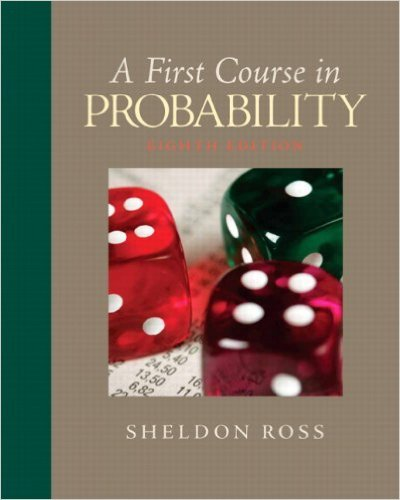×
Get Full Access to First Course In Probability - 8 Edition - Chapter 7 - Problem 7.42
Get Full Access to First Course In Probability - 8 Edition - Chapter 7 - Problem 7.42

×

# A group of 20 people consisting of 10 men and10 women isISBN: 9780136033134 163

## Solution for problem 7.42 Chapter 7

First Course in Probability | 8th Edition

• Textbook Solutions
• 2901 Step-by-step solutions solved by professors and subject experts
• Get 24/7 help from StudySoup virtual teaching assistantsFirst Course in Probability | 8th Edition

4 5 1 278 Reviews
29
0
Problem 7.42

A group of 20 people consisting of 10 men and10 women is randomly arranged into 10 pairs of2 each. Compute the expectation and variance ofthe number of pairs that consist of a man and awoman. Now suppose the 20 people consist of 10married couples. Compute the mean and varianceof the number of married couples that are pairedtogether.

Step-by-Step Solution:
Step 1 of 3

X, Y Bivariate Random Variables f(u1, 2 ) is density of (X,Y) ∫ ( ) density of X ∫ ( ) density of Y X, Y are independent if f(u1, 2 = f1(u1 2 (u2) If X1, ,2…….X are nndependent Var(X 1 ……..X ) = n + …….. We want to produce to specification “m” “m” = length of a part, content of juice can etc We want to check at the end of the assembly line, if we are meeting the quality of the product (Quality-Control) Process: We choose a sample of size “n” from the product X 1 X 2 …….X n are independent random variables. They have the same distribution. Look at the average of the If X is a random variable then Var(aX) Var( ) = = = ( We

Step 2 of 3

Step 3 of 3

##### ISBN: 9780136033134

Unlock Textbook Solution